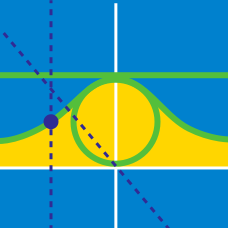Calculus

# Parametric Equations - Arc Length

The location of a dot $$P$$ at a given time $$t$$ in the $$xy$$ plane is given by $$(x,y) = (t - \sin t, 1 - \cos t)$$. What is the distance traveled by $$P$$ in the interval $$0 \leq t \leq 2\pi$$?

What is the length of the curve parametrized by the equations $\begin{array} \displaystyle x=e^{2t}\cos t, & y=e^{2t}\sin t,\end{array}$ in the domain $$0 \leq t \leq 4 ?$$

If $$x=4\sin^2 t$$ and $$y=4\cos^2 t,$$ what is the distance traveled by the point $$P=(x,y)$$ during the time interval $$0 \leq t \leq 5\pi?$$

Given the curve $$H(t) = \frac{2}{3} (t+4)^{3/2}$$, the arc length of the graph between $$t=4$$ and $$t=12$$ can be expressed in the form $\frac {a\sqrt{b}}{c} - d$ where $$a$$, $$b$$, $$c$$, and $$d$$ are positive integers, $$a$$ and $$c$$ are coprime, and $$b$$ is not divisible by the square of any prime. What is $$a+b+c+d$$?

Given the curve defined by $$x = t^3$$ and $$y = t^2,$$ what is the length of the curve from $$t=0$$ to $$t= 10?$$

×